# Divide

Divide area of rectangles with dimensions 32m and 10m by the ratio 7: 9. What area corresponds to a smaller section?

S1 =  140 m2
S2 =  180 m2

### Step-by-step explanation: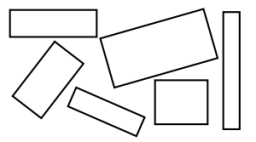Did you find an error or inaccuracy? Feel free to write us. Thank you!Tips to related online calculators
Need help to calculate sum, simplify or multiply fractions? Try our fraction calculator.
Check out our ratio calculator.

#### You need to know the following knowledge to solve this word math problem:

We encourage you to watch this tutorial video on this math problem:

## Related math problems and questions:

• Two rectangles 2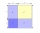A square of area 36 cm2 is cut out to make two rectangles. A and B The area of area A to area B is 2 : 1 Find the dimensions of rectangles A and B.
• ScaleThe swimming pool is long 110 m and 30 m wide. The plan of the city is shown as a rectangle with an area 8.25 cm2. What scale is the city plan?
• GardenThe rectangular garden has dimensions of 27 m and 30 m. Peter and Katka split it in a ratio of 4:5. How many square meters did Katkin measure part of the garden?
• RectanglesThe perimeter of a rectangle is 90 m. Divide it into three rectangles, the shorter side has all three rectangles the same, their longer sides are three consecutive natural numbers. What is the dimensions of each rectangle?
• RectanglesHow many different rectangles can be made from 60 square tiles of 1 m square? Find the dimensions of these rectangles.
• Two rectanglesI cut out two rectangles with 54 cm², 90 cm². Their sides are expressed in whole centimeters. If I put these rectangles together I get a rectangle with an area of 144 cm2. What dimensions can this large rectangle have? Write all options. Explain your calc
• Rectangles - sidesOne side of the rectangle is 10 cm longer than second. Shortens longer side by 6 cm and extend shorter by 14 cm increases the area of the rectangle by 130 cm2. What are the dimensions of the original rectangle?
• Three rectangles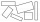Some wire is used to make 3 rectangles: A, B, and C. Rectangle B's dimensions are 3/5 cm larger than Rectangle A's dimensions, and Rectangle C's dimensions are again 3/5 cm larger than Rectangle B's dimensions. Rectangle A is 2 cm by 3 1/5 cm. What is the
• Rectangle 35Find the area of a rectangle when the diagonal is equal to 30 cms and the width is double the length.
• Divide moneyDivide 1200 USD at a ratio of 1:2:3:4:5:6:9:10
• Rectangle 3-4-5The sides of the rectangle are in a ratio of 3:4. The length of the rectangle diagonal is 20 cm. Calculate the content of the rectangle.
• Three gardensIs it possible to divide a plot of land with an area of 920 m2 into three gardens in a ratio of 4: 2: 3 so that their areas are only whole multiples of square meters?
• Ratio - rectangleThe rectangle has dimensions 6 cm and 9 cm. How many times increases area and its perimeter, when its dimensions increase in the ratio 5:3?
• Rectangular land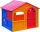On a rectangular land with dimensions of 35 m and 18.5 m is a house with a square floor plan with a side of 14 m. What % of the land is not occupied?
• FloorThe floor area of ​​the room is 31 m2 and has a width of 4.3 m. How many centimeters of circumference measured the floor on the map at the scale 1:75?
• ExpansionIf one side of the rectangle is larger 4-times and second 2-times, what percentage increases the area of rectangle?
• Rectangle - desc circle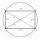Length of the sides of the rectangle are at a ratio 1: 3 . Radius of the circle circumscribed to rectangle is 10 cm. Calculate the rectangle's perimeter.